## Introduction

The term ‘Geometry’ is the English equivalent of the Greek word ‘Geometron’.

‘Geo’ means the Earth and ‘metron’ means Measurement. So, geometry means the measurement of the earth.

According to historians, the geometrical ideas came into picture when the boundaries of cultivated lands had to be marked without any confusion or complaint. Look around. You will find objects of different shapes in your room.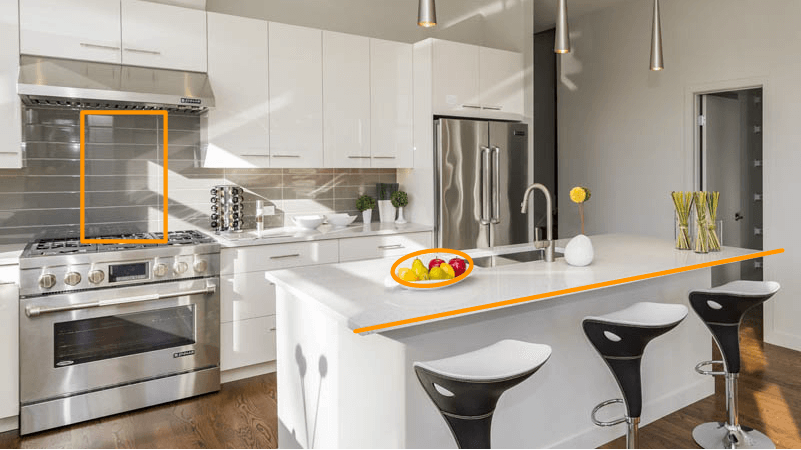All the objects around us are three dimensional. These objects may have sides, edges, surfaces, or corners which are basically a point, a line, a two-dimensional figure, or a curve.

In our daily life, we use geometrical ideas in many areas. Let us watch this video to know more about that.

Do you know geometry is around us when we step outside to go somewhere? For example, when you are travelling in a car or are on your way to school in a bus, look at the roads. You will notice that the two sides of the road are parallel and do not meet. This is a property of parallel lines.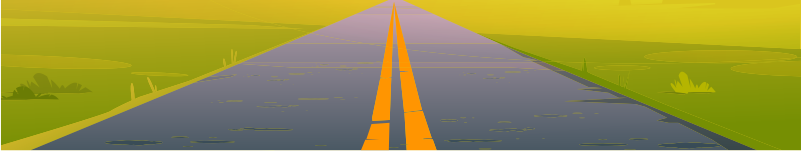#### Concepts

The chapter ‘Basic Geometrical Ideas’ covers the following concepts:

#### Basic Geometrical Shapes

Each corner where two edges of a square sheet meet is a point. Also, a tiny dot marked on a sheet of paper using the sharp tip of a pencil will give an idea of a point.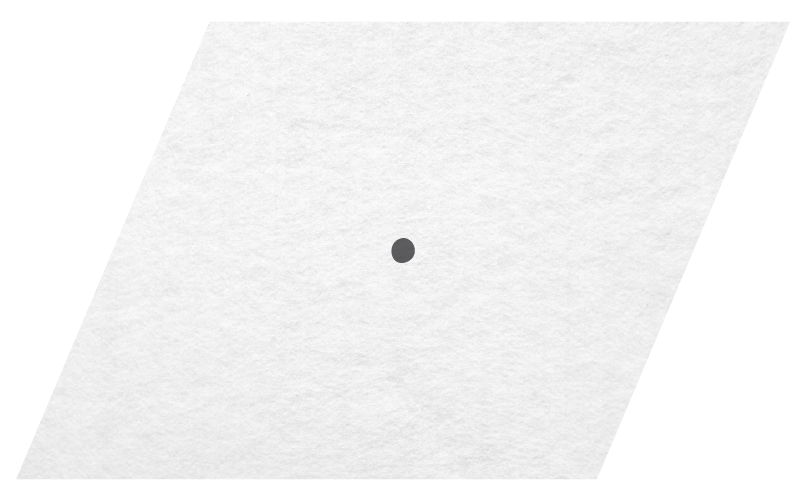A point determines a location. We can mark plenty of points on a sheet of paper.

In order to distinguish them, we need to name the points. So, they are denoted by a single capital letter like A, B, C, etc.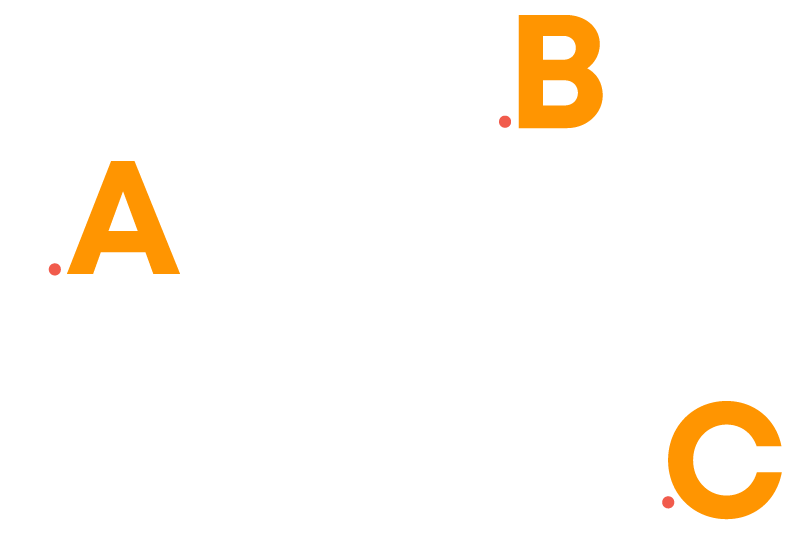These points will be read as point A, point B, and point C, etc. Now, consider the point A and the point B. Let us try to join them.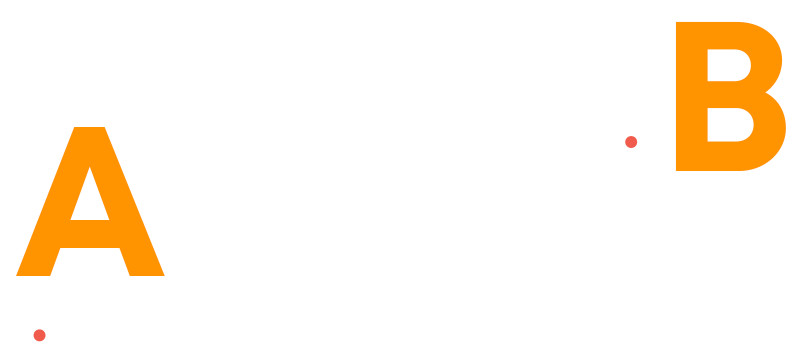This gives the idea of a line segment. As we see here, the line segment starts at the point A and ends at the point B. That means the segment does not go beyond the points A and B. So, the point A and the point B are called its endpoints.

Imagine that the line segment between A and B is extended beyond A in one direction and beyond B in the other direction without any end.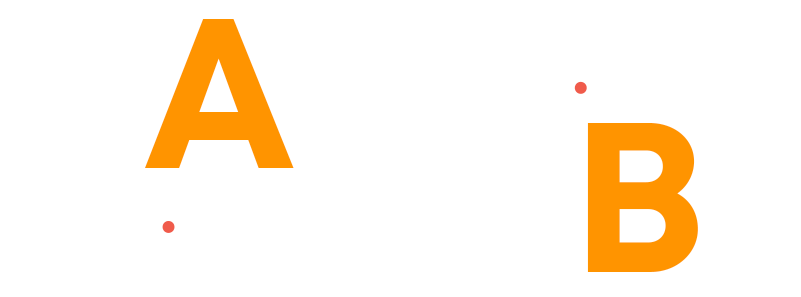This gives a model for a line. We can see here that the line is extending beyond A and B, endlessly.

Thus, the points A and B are two points on the line, but not its endpoints. A line extends beyond any point lying on it. So, no point can be an endpoint of a line.

What if we extend the line segment in only one direction?

Imagine that the line segment between A and B is extended beyond B in the same direction without any end.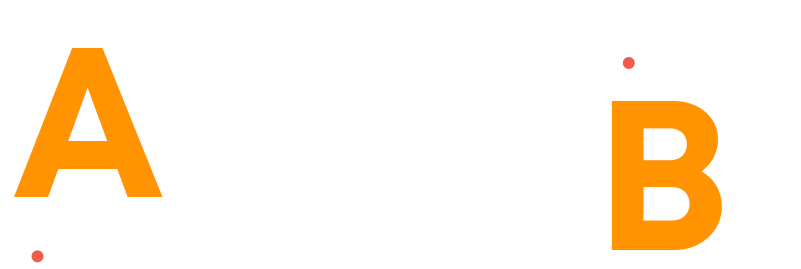This gives a model for a ray. We can see here that the ray is starting from the point A and extending beyond B, endlessly.

Thus, the point A is an endpoint (can also be called the initial point) of the ray and B is a point on the ray, but not an endpoint of the ray.

A ray has a fixed point from where it starts and extends beyond any other point lying on it. So, the starting point of the ray can be considered as its one endpoint. Thus, a ray can have only one endpoint.

We have learnt about the properties of lines, line segments and rays. Using these properties, we can identify them.

Suppose you are at a beach.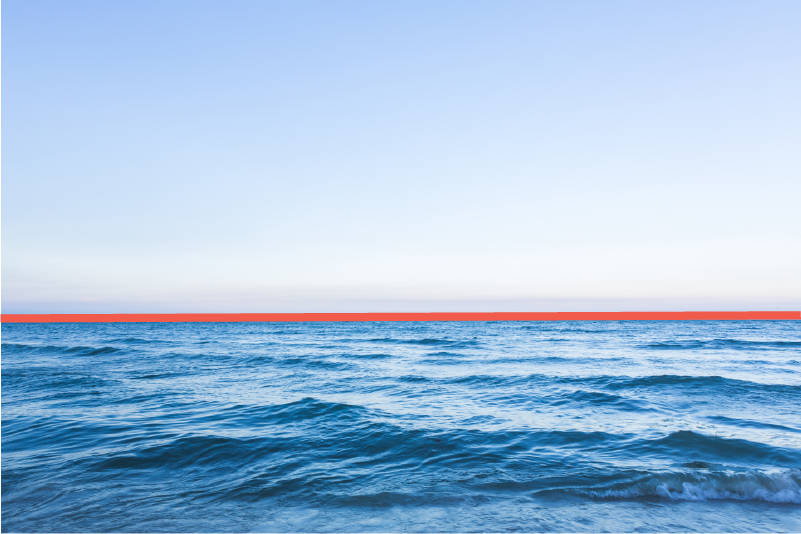You can see the edge between the sea and the sky. Can you measure the length of the edge? No. Does it have an endpoint? No, it does not have any endpoint. It extends endlessly in both directions. So, you cannot measure its length.

So, this is an example for a line. Thus, to identify a line, we need to check whether it is endless.

To identify a line segment, we need to check whether it has two endpoints. To identify a ray, we need to check whether it has an initial point and extends endlessly (only one endpoint).

Now let us learn how to name lines, line segments, and rays.

• A line segment is represented using its endpoints, with a bar over them. The line segment AB is denoted by AB or BA.
• A line is named using any two points on it with a double headed arrow over them. If M and N are any two points on a line, then the line can be represented as MN⃡.
• A ray is named using its initial point and any point on it with an arrow showing the direction from the initial point to the other point over the letters. AB is a ray starting at the point A and extending beyond the point B.

Now let us explore types of lines.

Parallel lines never meet, maintain a constant distance between them, and do not have a common point.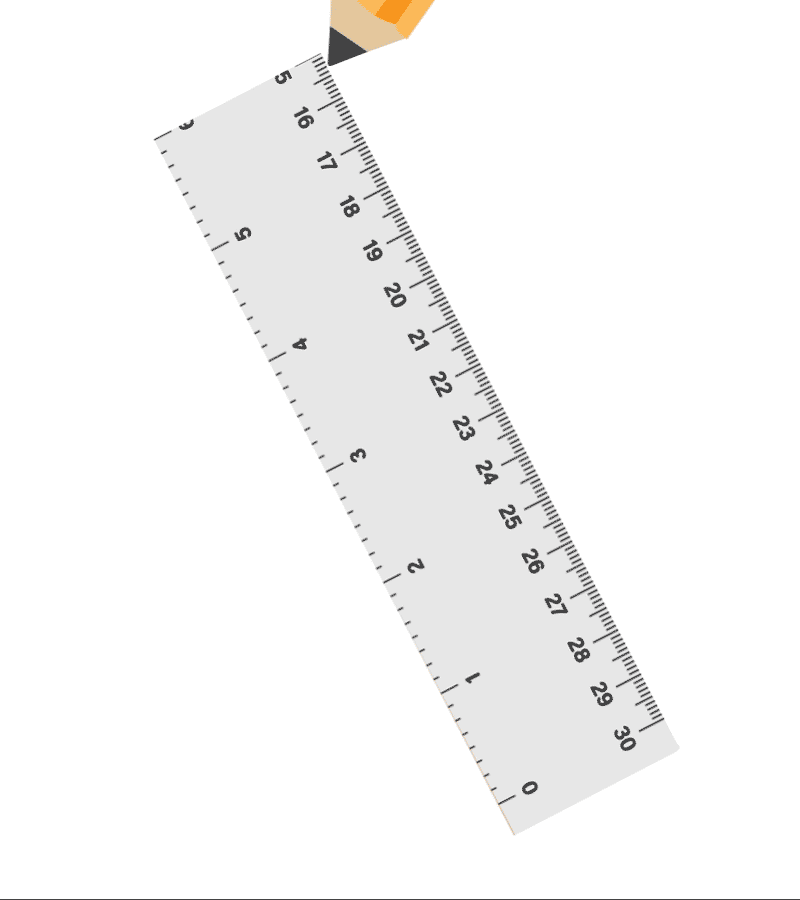Intersecting lines meet at a point (some maybe on extending), do not maintain a constant distance between them, and have a point in common, which is their point of intersection.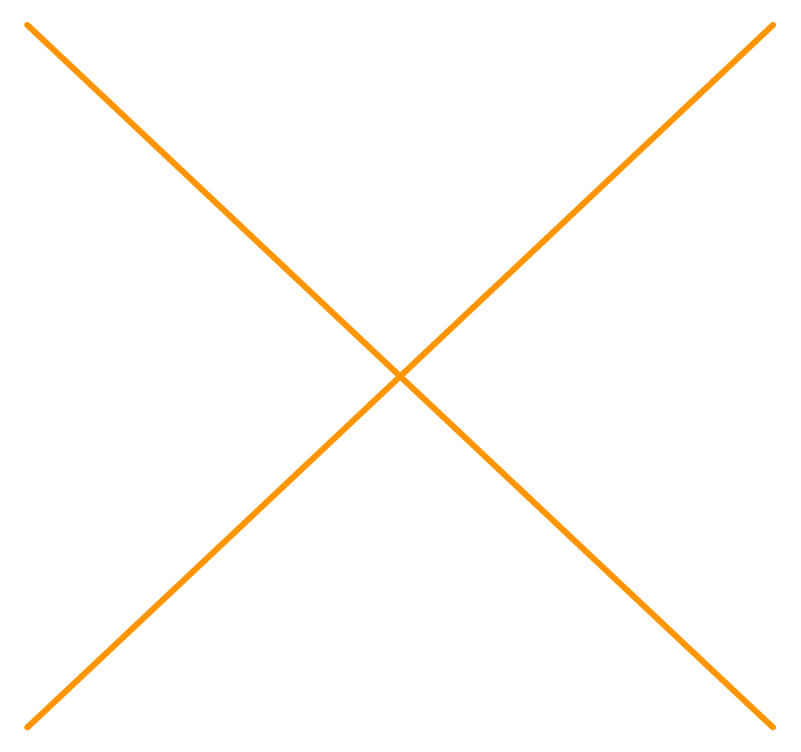Remember, two lines cannot meet at more than one point. The same pair of lines cannot be both parallel and intersecting.

If you observe few the picture below, you will find intersecting lines as well as parallel lines.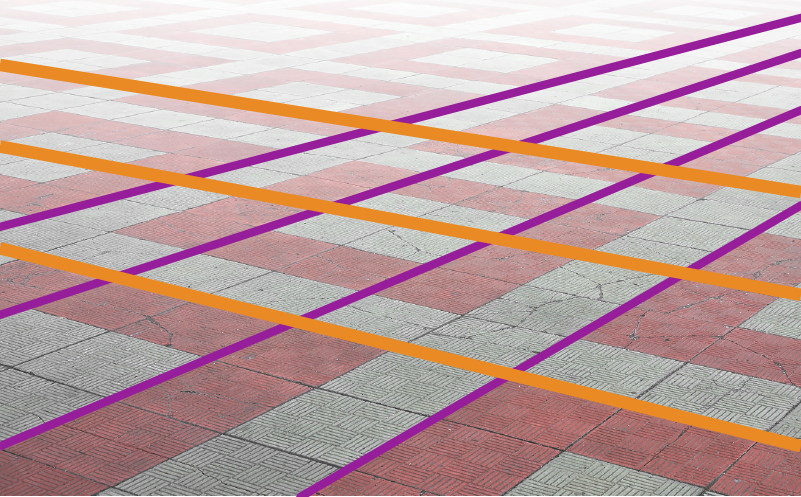To draw parallel lines, we should make sure that the distance between the lines is always constant.

To draw intersecting lines, draw a line and another line crossing it. Also, by drawing two lines which do not maintain a constant distance, we can draw intersecting lines, which will intersect only when extended.

You have already read about rays. An angle is made up of two rays starting from a common point. The two rays forming the angle are called the arms or sides of the angle. The common point is the vertex of the angle.

In specifying an angle, the vertex is always written as the middle letter. To show the angle, a small curve is used between the arms of the angle. Sometimes, an angle is named using only the vertex. For example, ∠POQ is the same as ∠QOP. ​Sometimes it can be represented as ∠O.

Consider the following angle.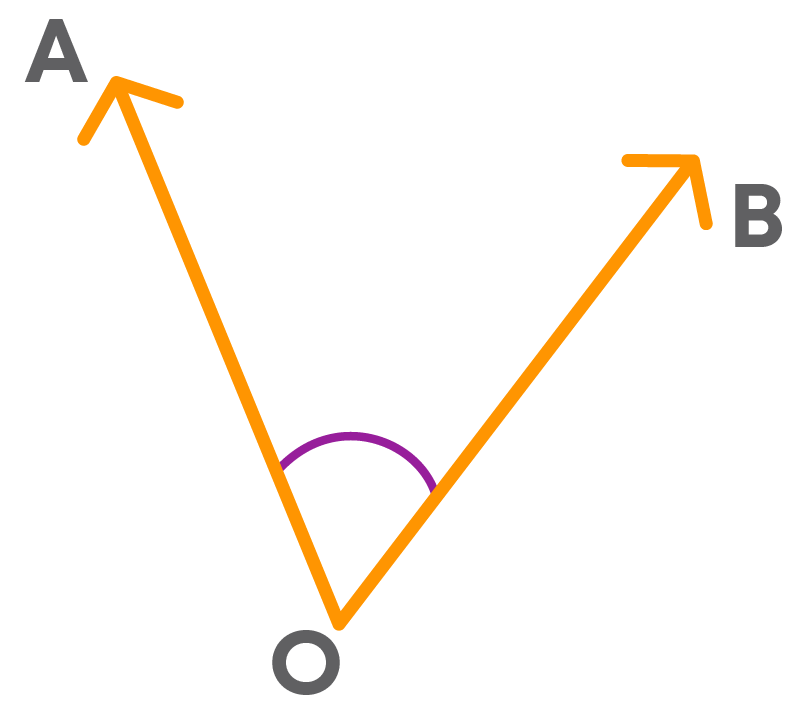Which are the arms of the angle? OA and OB. Can you identify the region between these two arms?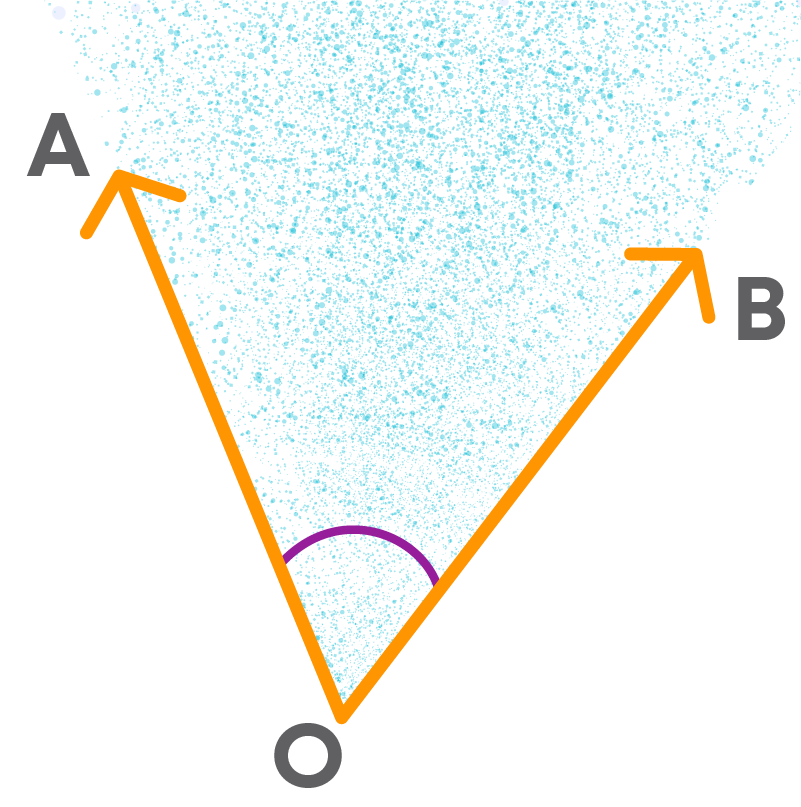The shaded portion is the region between the arms of the angle. This is known as the interior of the angle. Thus, the region enclosed between the two arms of an angle is called the interior of the angle and the rest of the plane is called its exterior.

Now, can you identify the points in the interior or exterior of the angle?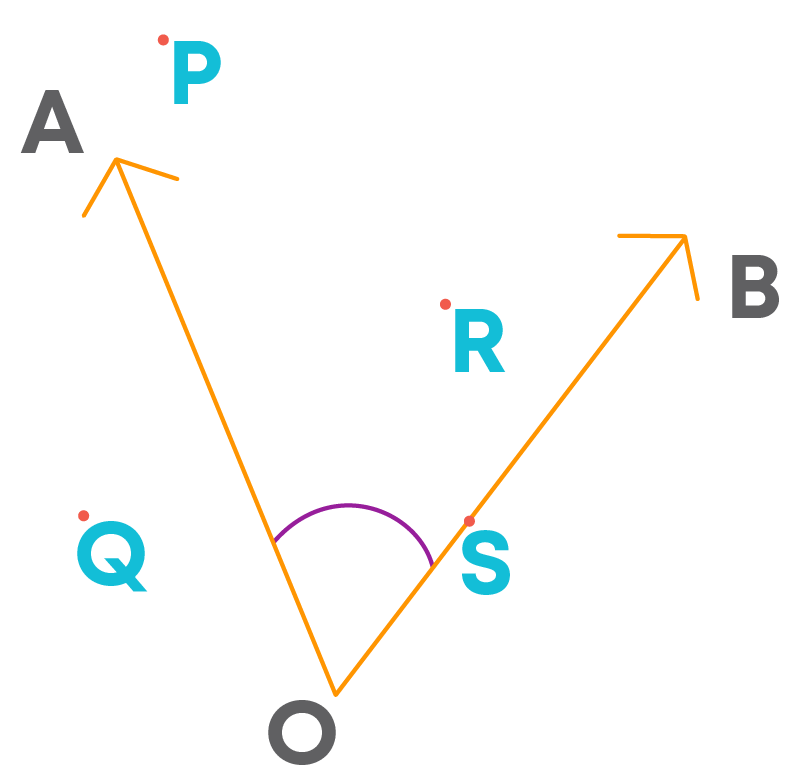#### Curves

‘Curve’ in everyday usage means “not straight”. In mathematics, a curve can be straight. For example, consider the following picture: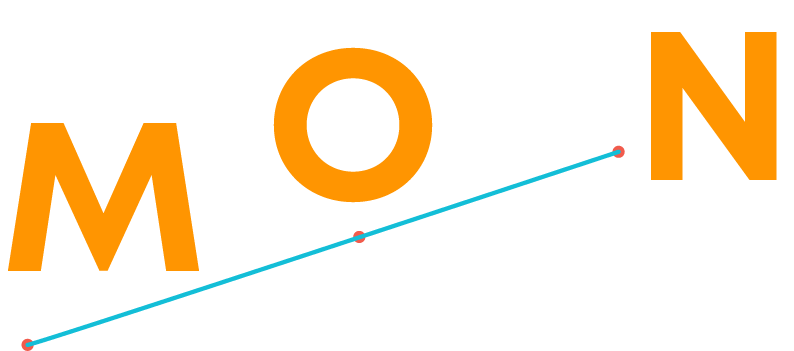It is a curve joining the points M, O and N, which is straight. Let us consider the curves given below.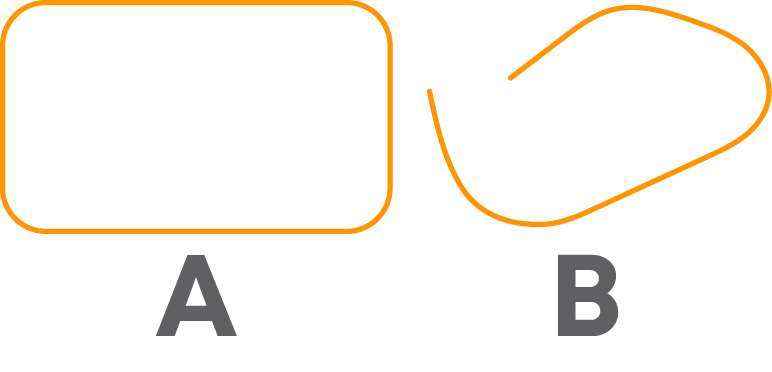Can you find distinct initial and endpoints in the curve A ?

No, for the curve A, the initial point and the endpoint is the same. The curve ends where it begins. Such a curve is called a closed curve. Is it the same in the case of the curve B?

No, for the curve B, there are two distinct points, at one point the curve begins and ends at another point. Such a curve is called an open curve.

Consider the curve A which is a closed curve. The area enclosed within the border of the closed curve is called its interior and the area beyond its border is called the exterior of the curve.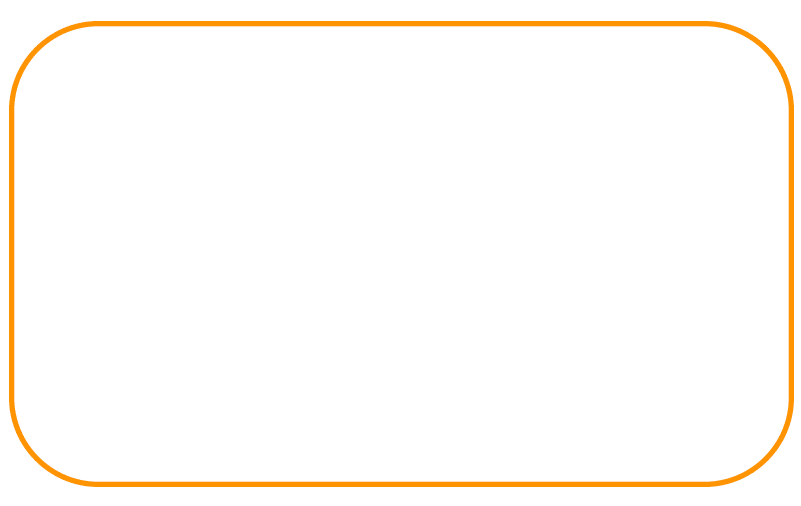The shaded region is the interior of the curve. The border is its boundary and the remaining region is its exterior.

Thus, a closed curve has three parts:

• 1. Interior
• 2. Exterior
• 3. Boundary

Consider: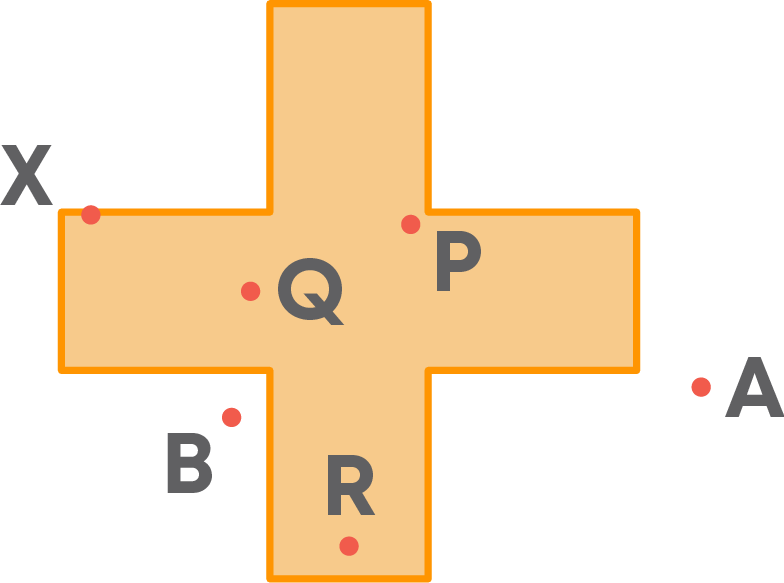• The points P, Q and R are in the interior of the curve.
• The points A and B are in the exterior of the curve.
• The point X is on the boundary of the curve.

#### Polygons

We know that a closed curve can have line segments as well as curved lines as parts of its boundary. Also, we can draw closed curves made up of only line segments. Such a figure is called a polygon. So, a figure is a polygon if it is a closed figure made up entirely of line segments.

Note: Here, the words ‘figure’ and ‘curve’ refer to the same thing. Consider the following polygon.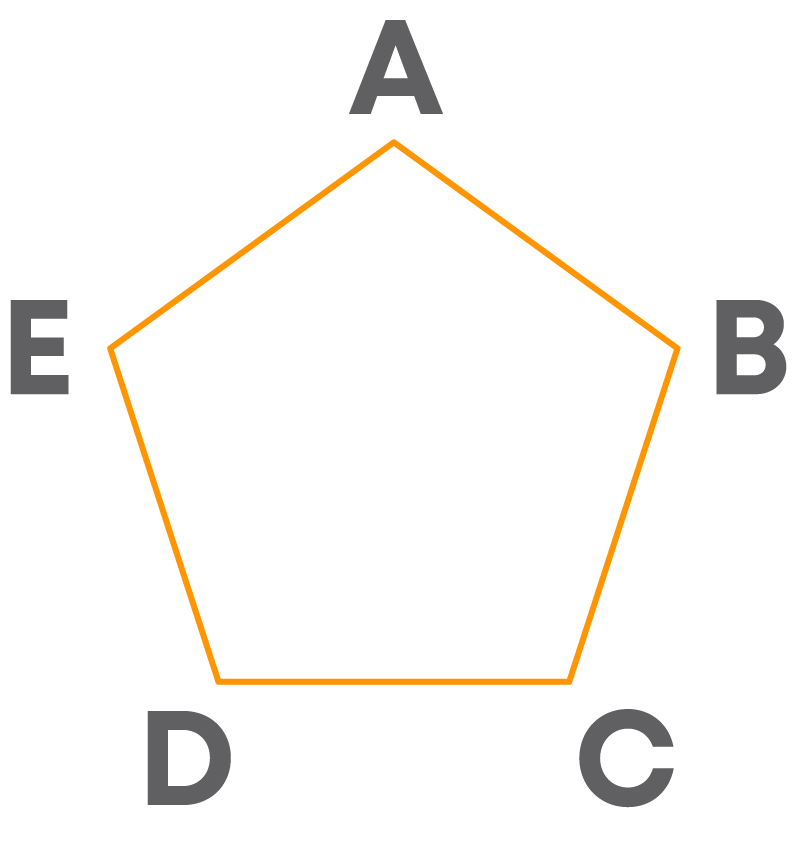Can you name the line segments forming the polygon ABCDE? The line segments AB, BC, CD, DE, and AE form the polygon ABCDE. These line segments forming the polygon are called its sides.

Do you observe that every pair of sides of the polygon meet at some point? The following table gives you the list.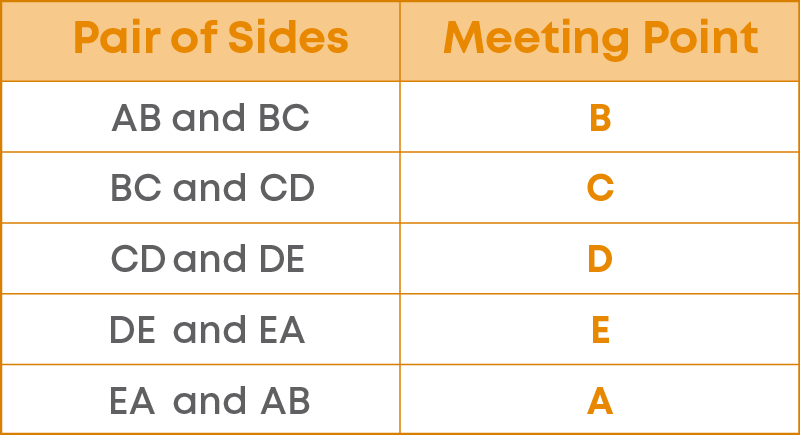These points where two sides of the polygon meet are called its vertices.‘Vertex' is the singular form of ‘vertices.’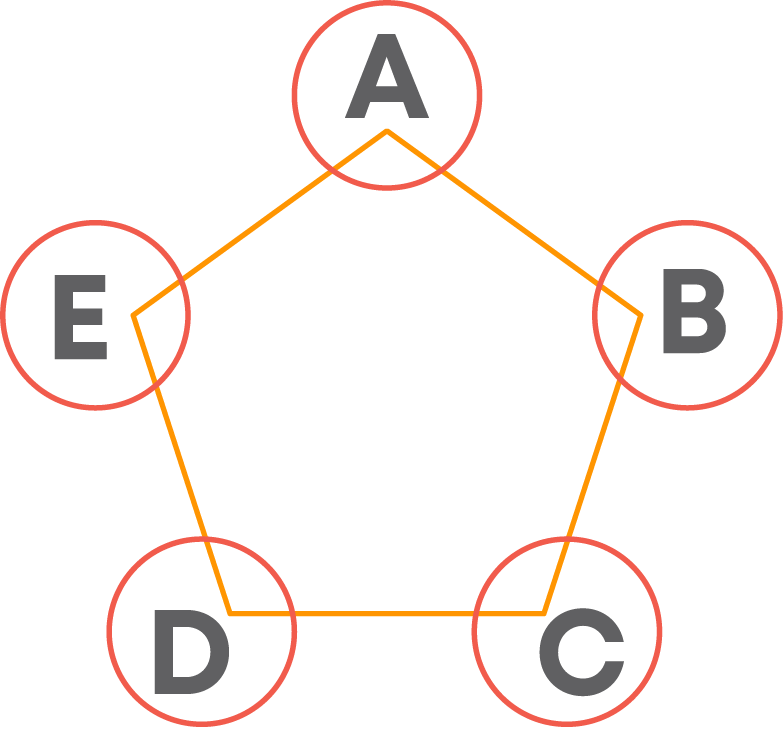Any two sides with a common vertex are called adjacent sides of a polygon. The endpoints of the same side of a polygon are called adjacent vertices.

We can see that at each vertex of the polygon, an angle is formed.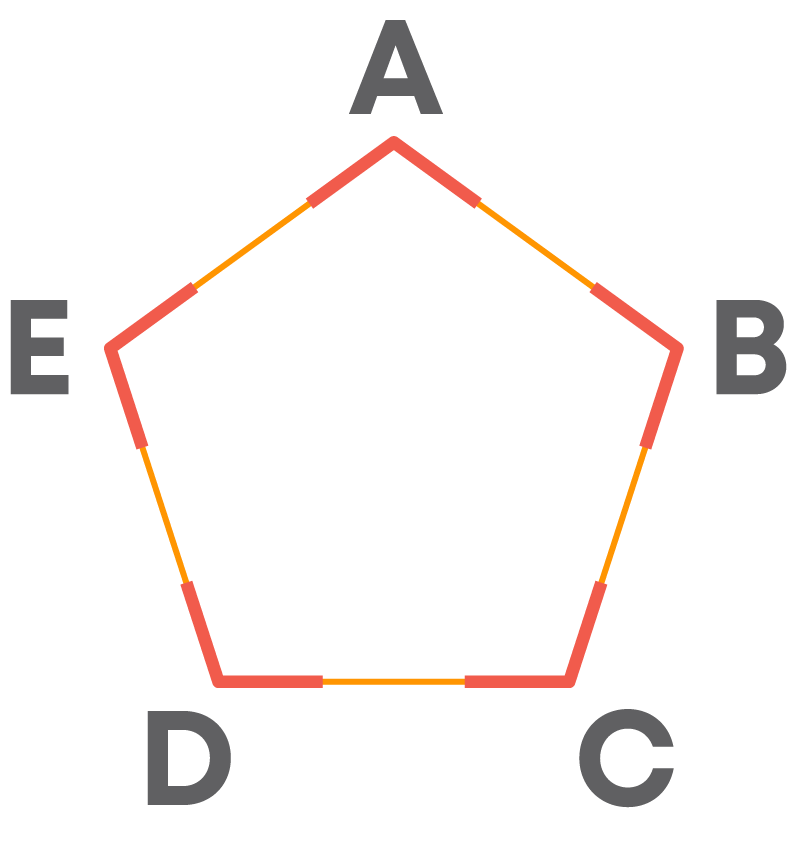We see that, the line segments AD and AC can be drawn with A as one of the endpoints. These line segments are called diagonals. Thus, a diagonal of a polygon connects two vertices of the polygon which are not adjacent.

So, let us see the other diagonals of the polygon ABCDE.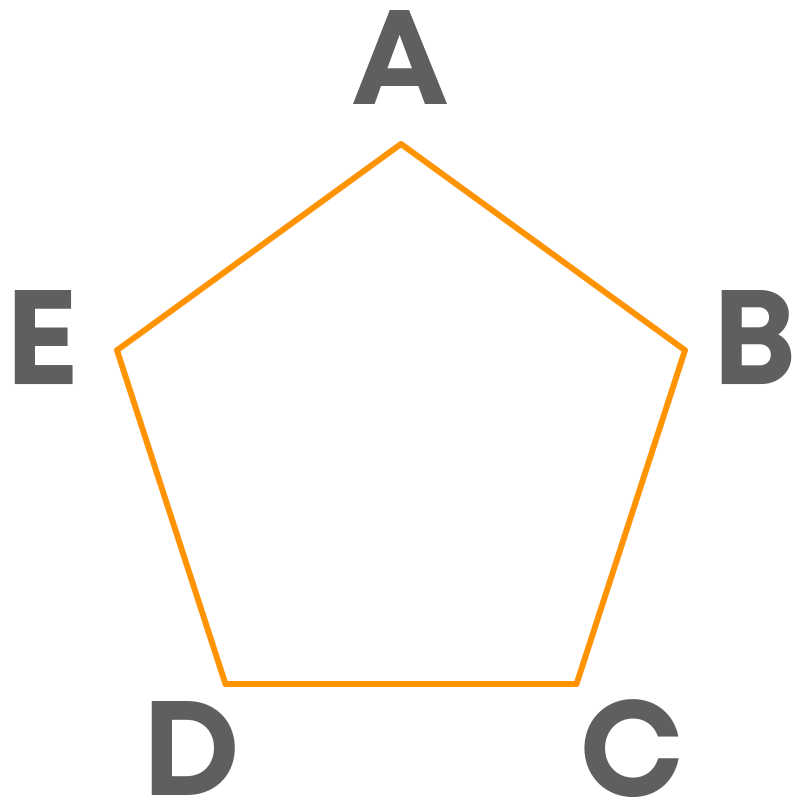A minimum of three line segments are required to form a polygon.What is this shape called? This is called a triangle. We see that a triangle is a closed curve and is made up of three line segments.

So, a triangle is a three-sided polygon. In fact, it is a polygon with the least number of sides.

What are the sides of the triangle given above? The line segments AB, BC and CA form the triangle ABC. So, they are the sides of the triangle. The points where the sides of the triangle meet are called the vertices of the triangle. So, A, B and C are the three vertices of the given triangle.

Do you observe that at these vertices, angles are formed? There are three angles.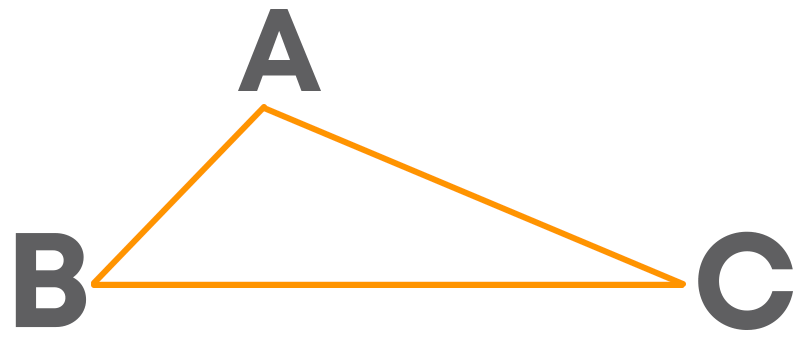A triangle has 3 sides, 3 angles and 3 vertices. The symbol Δ is used to represent a triangle.

Now, what is a closed curve made up of four line segments called? It is called a quadrilateral. What are the sides of the quadrilateral given below?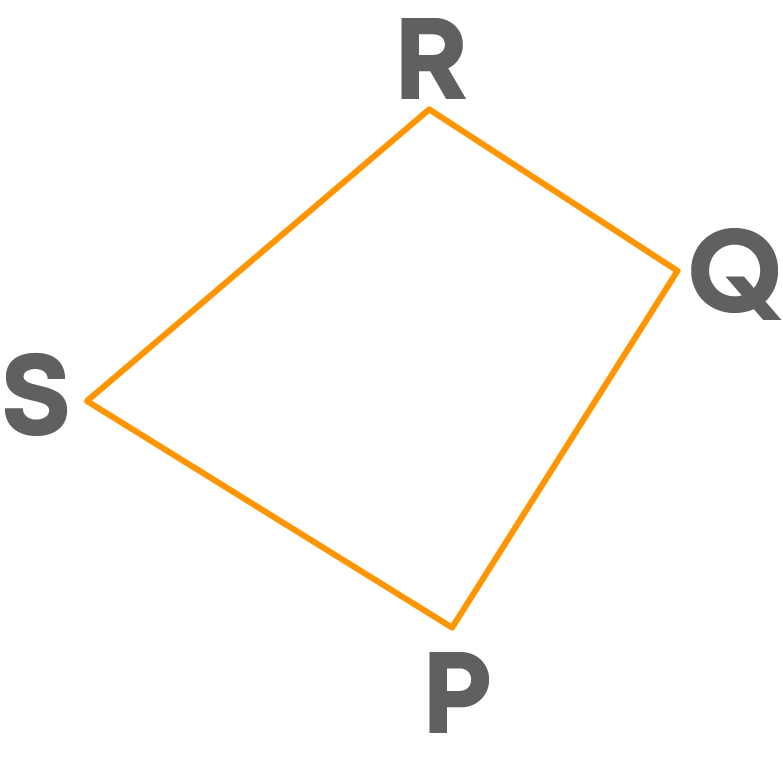The line segments PQ, QR, RS and SP form the quadrilateral PQRS. So, they are the sides of the quadrilateral. The points where these sides of the quadrilateral meet are called the vertices of the quadrilateral.

So, P, Q, R and S are the four vertices of the given quadrilateral. Do you observe that at these vertices, the angles are formed?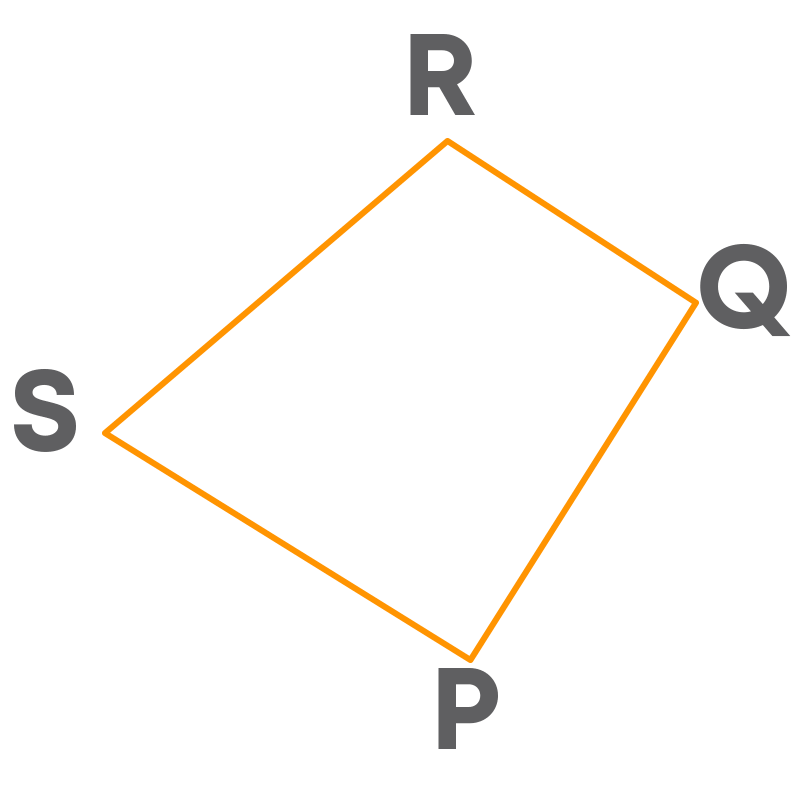So, how many angles are there? There are four angles. So, a quadrilateral has four sides, four vertices and four angles.

We know that a diagonal of a polygon connects two vertices of the polygon which are not adjacent.

Here, which are the pairs of vertices which are not adjacent?

• P and R
• Q and S

If we connect P to R and Q to S, we get the diagonals of the quadrilaterals.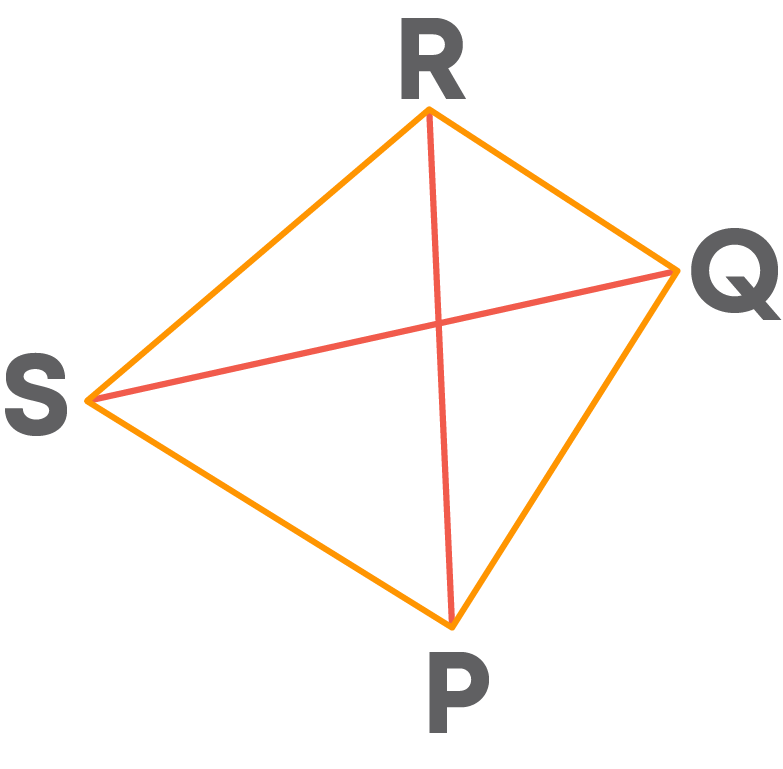PR and QS are the diagonals of the quadrilateral PQRS.

• A quadrilateral is named using the vertices written in a cyclic order.
• A pair of sides having one vertex in common is called a pair of adjacent sides.
• A pair of sides having no common vertex is called a pair of opposite sides.
• There are two pairs of opposite sides in a quadrilateral.
• A pair of angles formed at the endpoints of the same side is called a pair of adjacent angles.
• A pair of angles opposite to each other is called a pair of opposite angles.
• There are two pairs of opposite angles in a quadrilateral.

#### Circle

Take two pencils. Fix one of them vertically on a sheet of paper and the other one slantly to draw on the sheet, as shown below.What do you get? You get a circle. The point made by the tip of the fixed pencil is the centre of the circle obtained.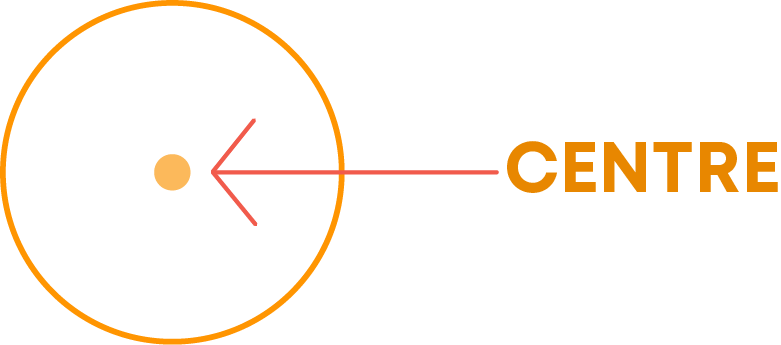While drawing the circle, did the distance between the tips of the two pencils alter? No, the distance between them was kept constant. This constant distance is called the radius of the circle.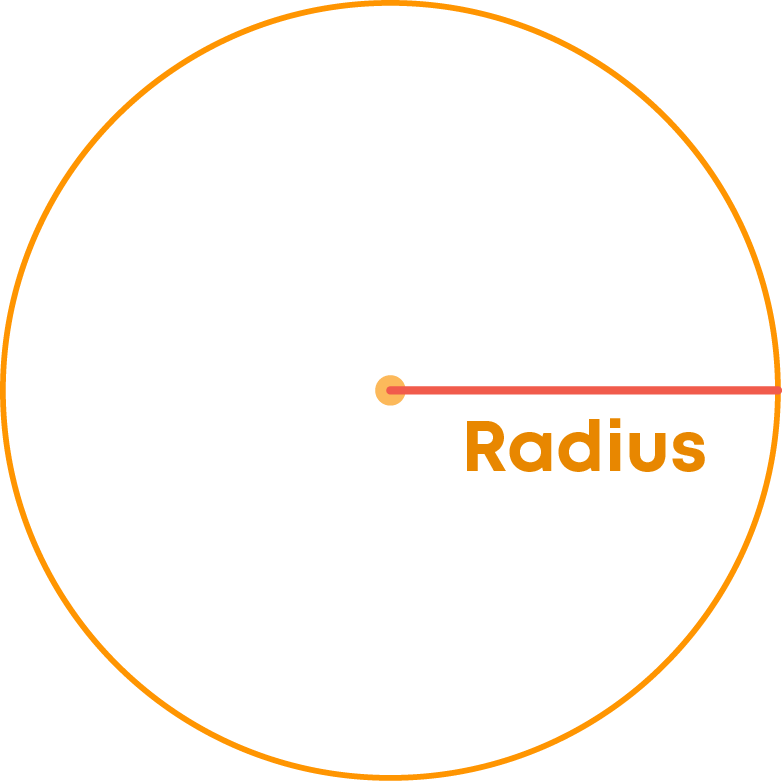We see that the radius is a line segment that connects the centre to a point on the circle. How many radii (the plural form of radius) can you draw here? We can draw many or infinite number of lines through a point. This means that from the centre of the circle, we can draw many radii.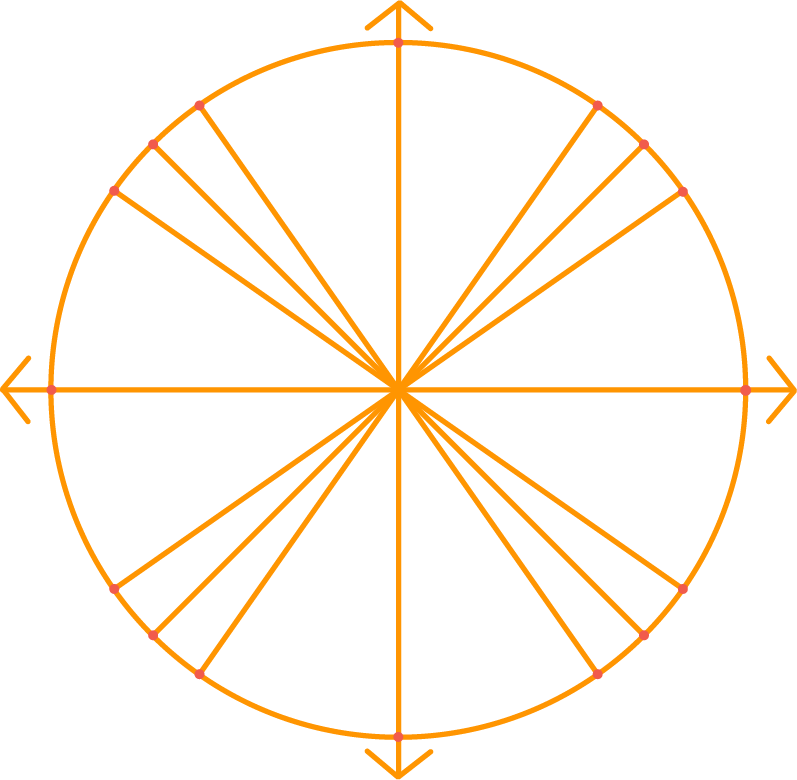Consider two radii forming a straight line.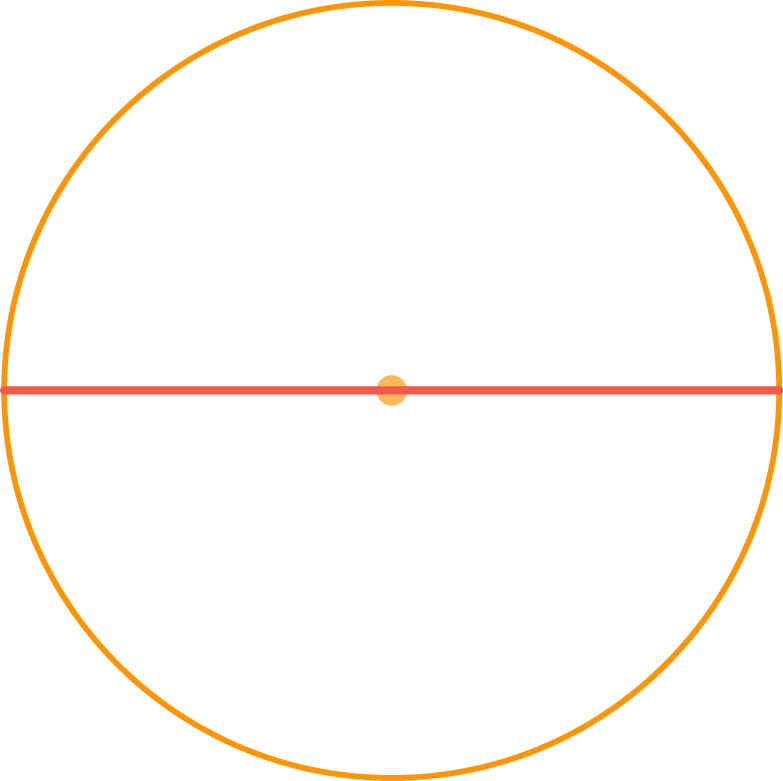These two radii together give a longer line segment which connects two points on the circle and it passes through the centre of the circle. This line segment is called a diameter of the circle. The figures below show different diameters of a circle.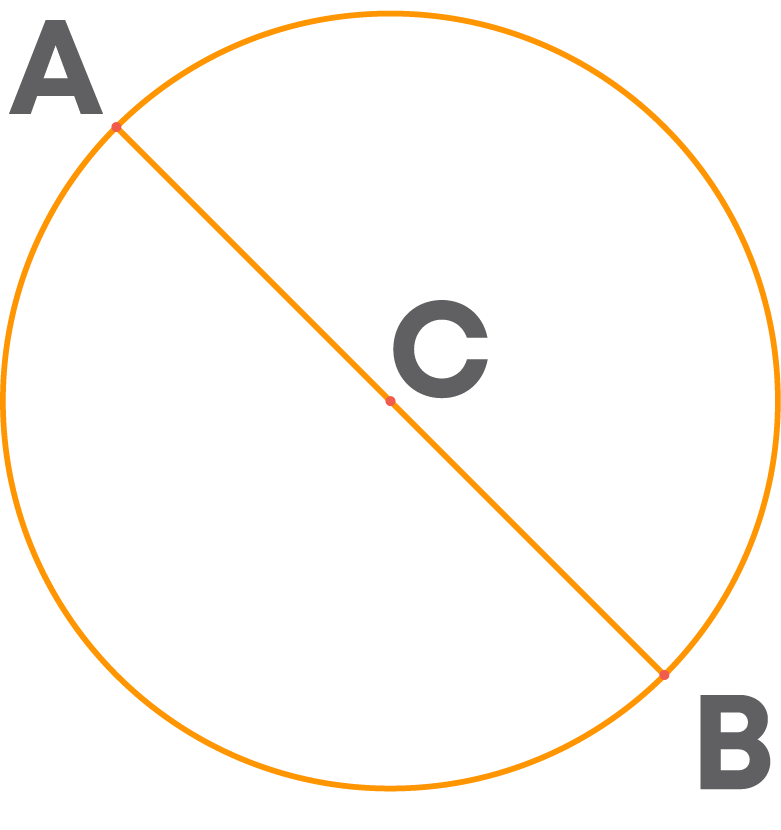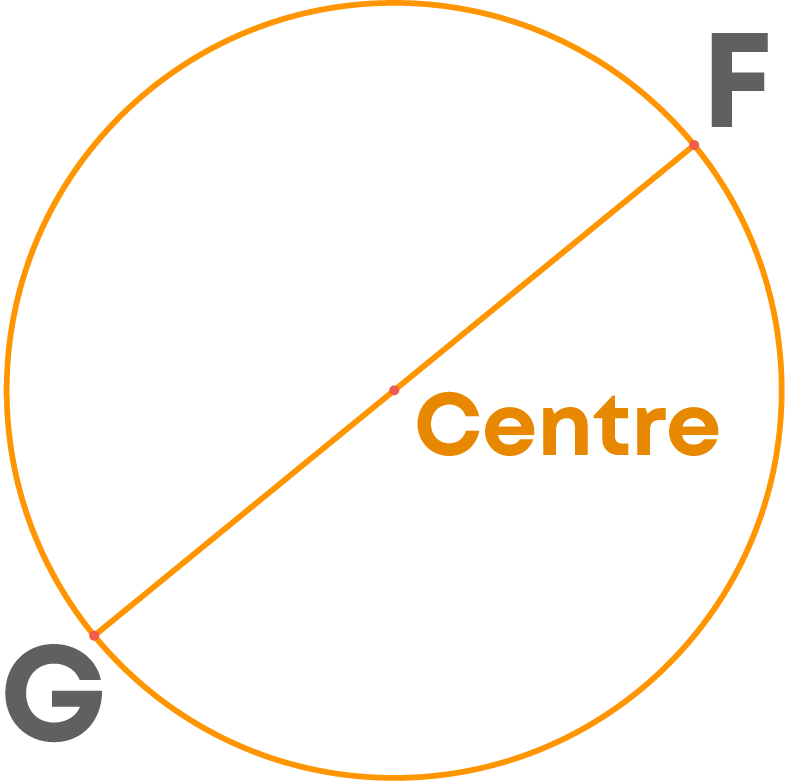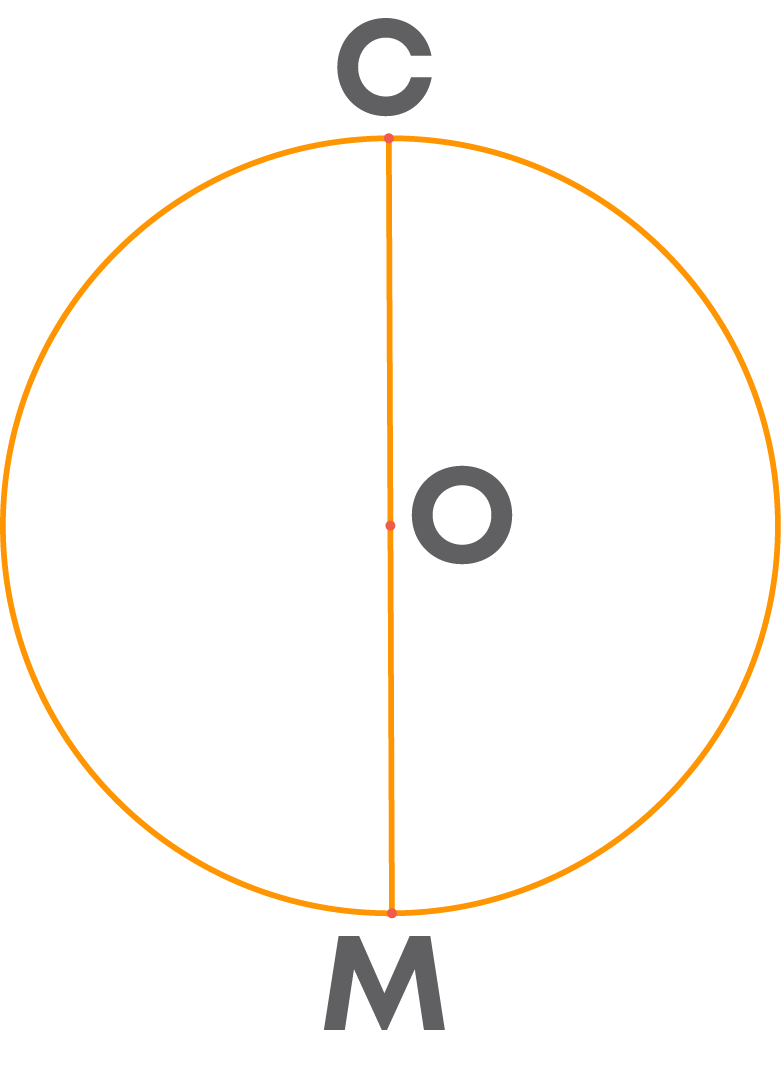Now, let us learn about some more parts of a circle.In the figure, we find two points A and B on the circle. The part of the circle between these two points is called an arc.

So, an arc is a part of a circle. Now, join the points A and B with a line segment.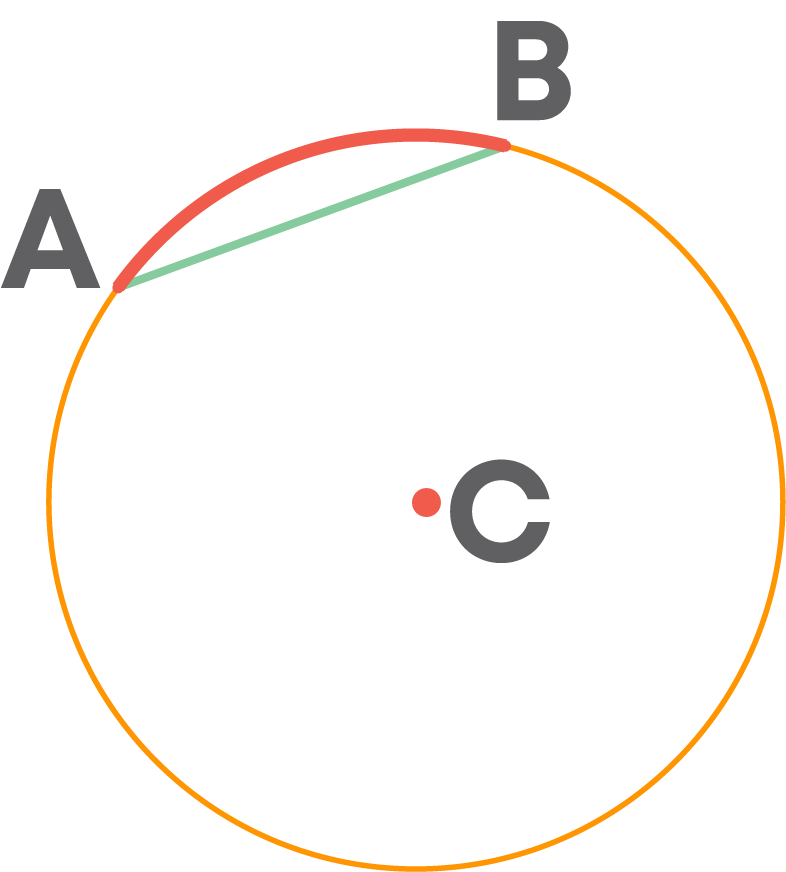We know that a diameter connects two points on a circle passing through the centre.

Is this line segment passing through the centre? No.

Here, AB is a line segment that connects A and B, but it does not pass through the centre. So, it is not a diameter. It is called a chord. We know that a circle is a closed curve. So, it has an interior.

Now, consider the part of the interior of the circle enclosed between the chord and the arc shown above.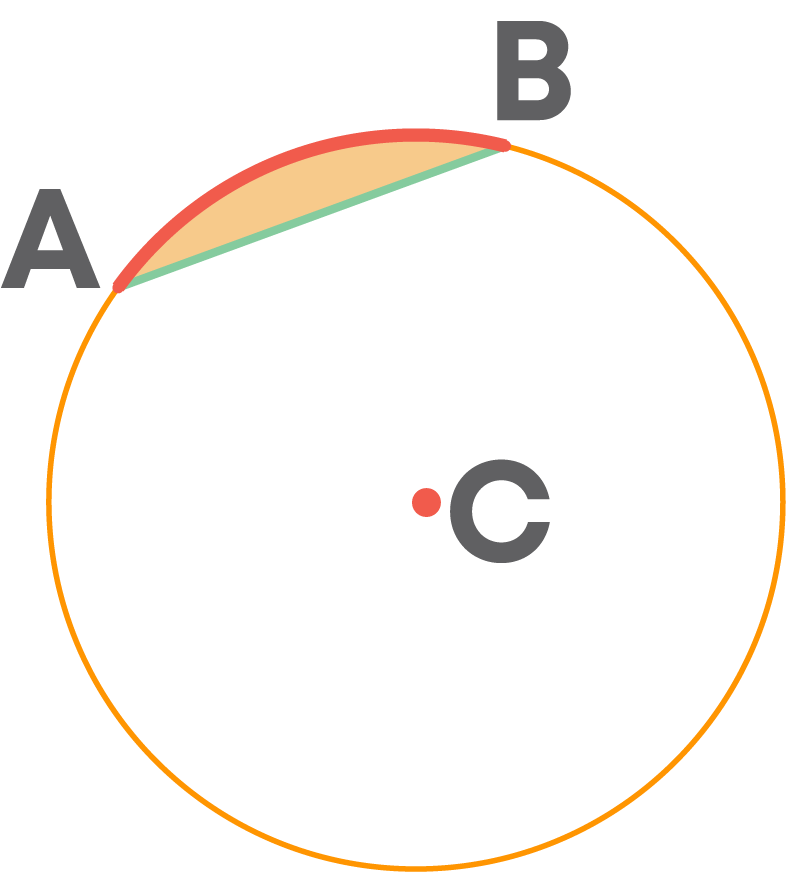This region is called a segment of the circle.

We can observe below that a part of the interior of the circle is enclosed between the two radii and the arc between the points A and B.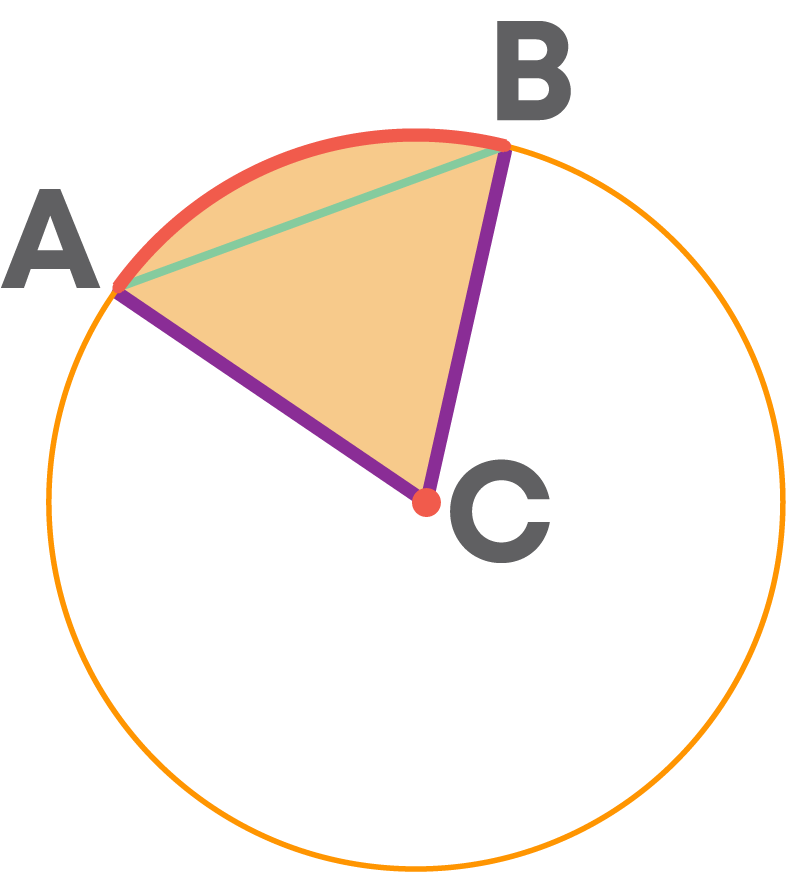This region is called a sector of the circle.

#### Common Errors

The following are topics in which students make common mistakes when dealing with basic geometry:

• 1. Intersecting lines
• 2. Representing a ray
• 3. Naming an angle
• 4. Circle

#### Intersecting Lines

Two lines which are not parallel, intersect at a point even if their point of intersection is not shown.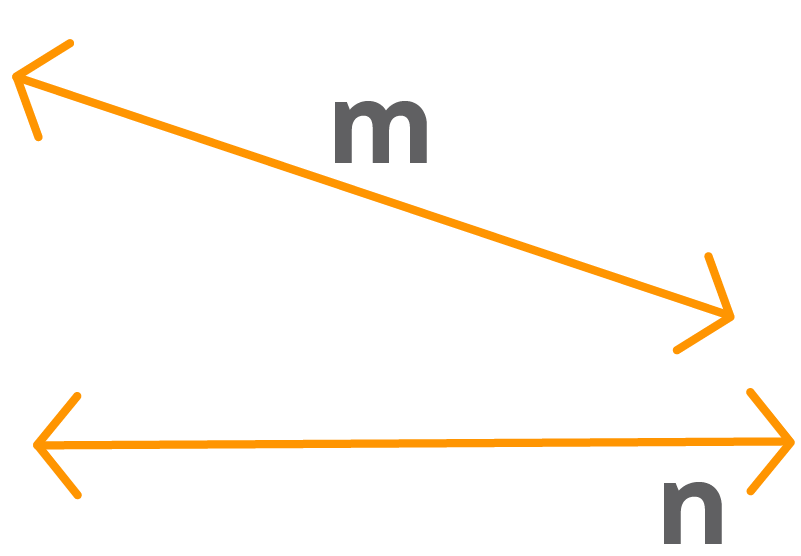In the given figure, the lines ‘m’ and ‘n’ are not parallel. Though their point of intersection is not shown in the figure, by extending the two lines on the right we can get their point of intersection.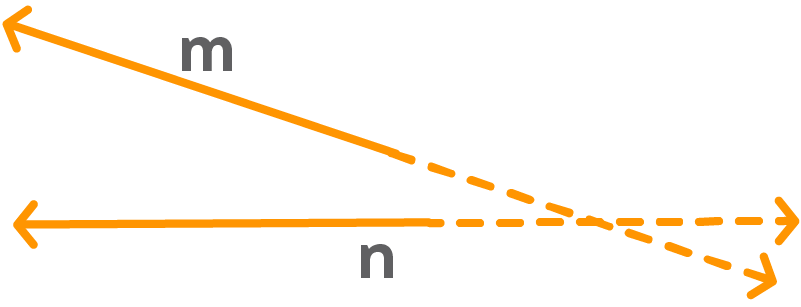#### Representing A Ray

To represent or name a ray we should always mention the initial point of the ray first. For example, ray AB and ray BA are not the same. Ray AB starts from the point A and ray BA starts from the point B. Ray AB can be represented as AB.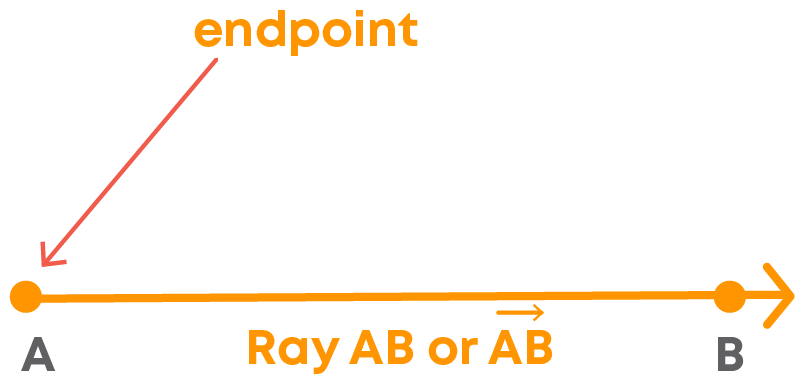The starting point of the arrow over the letters indicates the initial point of the ray. So, the same ray can be represented as BA⃖. But it is not the same as BA. BA and AB⃖ represent the ray BA.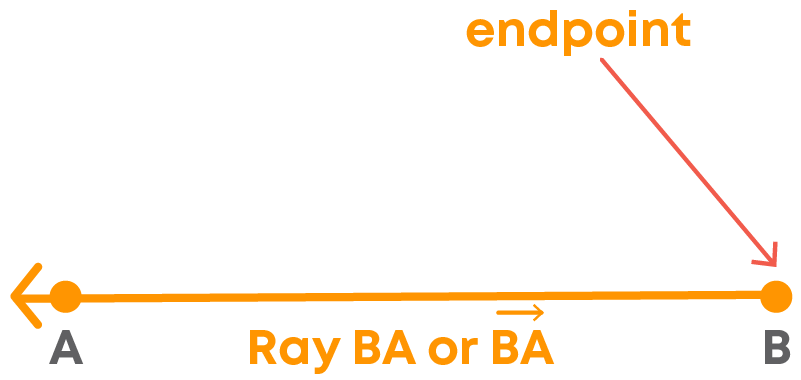#### Naming An Angle

If there are two or more angles formed at the same point, then mention all three letters to specify an angle. The vertex of the angle should be in the middle. Example: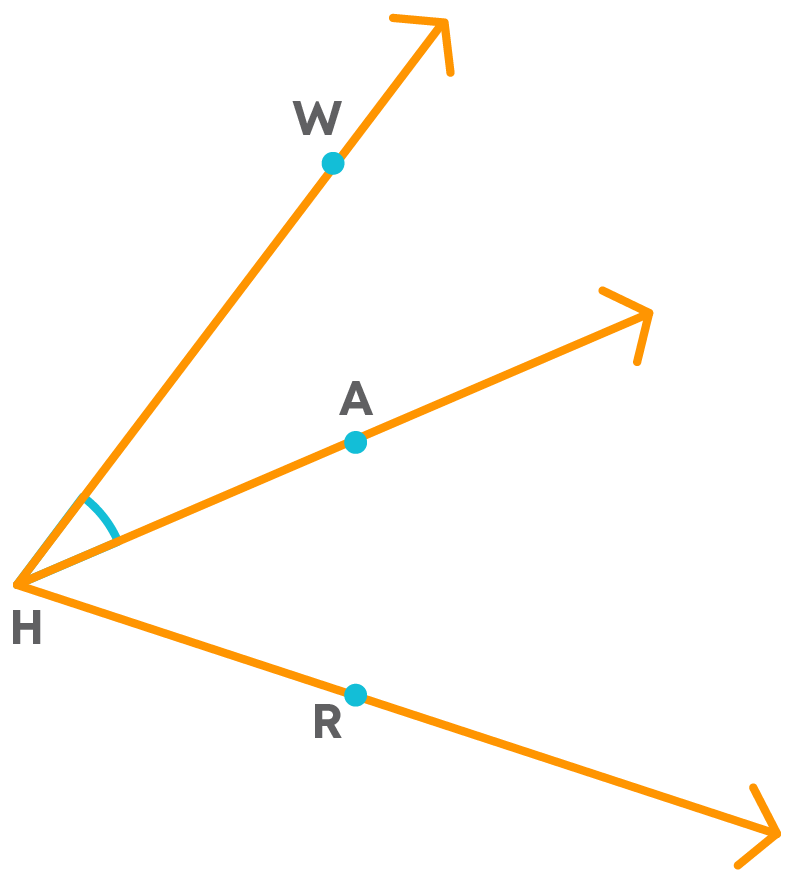In the given figure, there are three angles formed at H. So, to specify the marked angle, instead of writing ∠H, we should write ∠WHA or ∠AHW.

#### Circle

When we say, ‘a circle’, it is just the boundary, but not the interior of the circle. Consider a coin.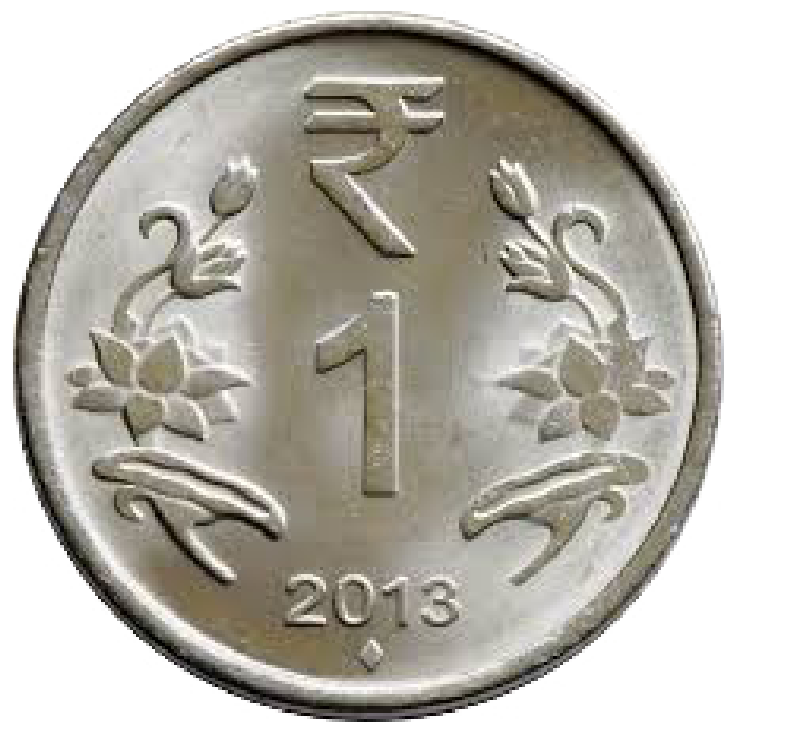A coin is not a circle. We can just say a coin has a circular shape.

i.e., its boundary is a circle.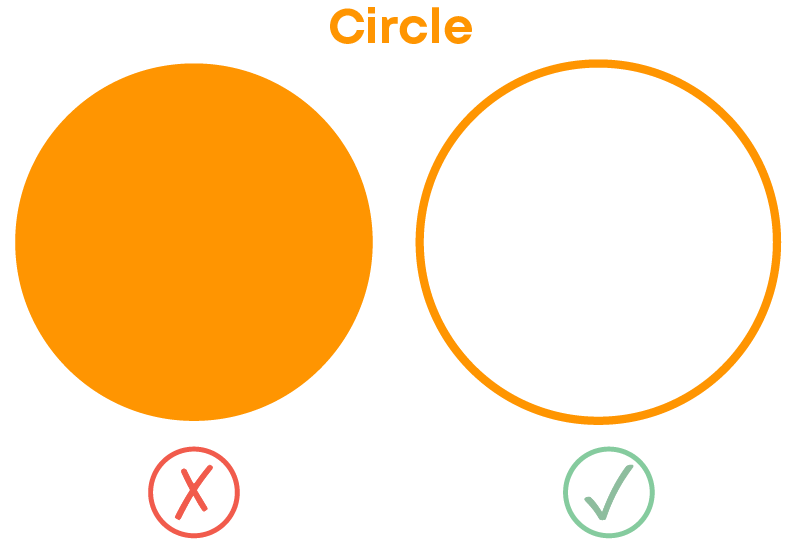#### Conclusion

You have learnt the basics of geometric shapes. Can you solve this puzzle?

Match the animals in the first column with the shapes used to make them in the second column. An example is done for your reference.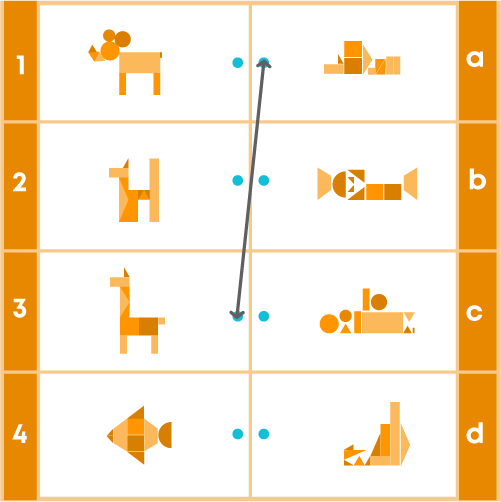#### Arpana

Author

Arpana is an education specialist with years of teaching Math and developing content. She previously worked as a freelance content developer, developing lesson plans for a reputed publisher of text books and content for various educational companies. She is an enthusiastic teacher who has taught students from India, the UK, and New Zealand.